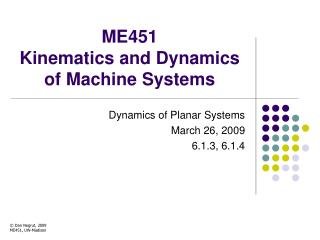DownloadDownload PresentationME451 Kinematics and Dynamics of Machine Systems

# ME451 Kinematics and Dynamics of Machine Systems

Download Presentation## ME451 Kinematics and Dynamics of Machine Systems

- - - - - - - - - - - - - - - - - - - - - - - - - - - E N D - - - - - - - - - - - - - - - - - - - - - - - - - - -
##### Presentation Transcript

1. ME451 Kinematics and Dynamics of Machine Systems Dynamics of Planar Systems March 26, 2009 6.1.3, 6.1.4 © Dan Negrut, 2009ME451, UW-Madison

2. Before we get started… • Last Time • Focused on deriving the “ma=F” equivalent that governs the time evolution of a 2D rigid body in planar motion • We started from “scratch” • The assumptions used: • Body is rigid • Working with centroidal body reference frame to simplify form of the equations of motion (EOM) • Today • Finish the derivation • Look at an example • Analyze inertia properties of rigid bodies 2

3. Deriving Newton’s Equations for a body with planar motion • NOTE: • You should be able to derive Newton’s equations for a planar rigid body on your own (closed books) • Overall, the book does a very good job in explaining the derivation the equations of motion (EOM) for a rigid body • No notes last time, the material is straight out of the book (page 200) 3

4. Inertia Properties of Rigid Bodies • Issues discussed: • Determining location of the center of mass (centroid) of a rigid body • Parallel axis theorem • Mass moment of inertia of composite bodies • Location of centroid for a rigid body with a symmetry axis 5

5. Location of the Center of Mass • By definition the centroid is that point of a body for which, when you place a reference frame at that point and compute a certain integral in relation to that reference frame, the integral vanishes: Q: How can I determine the location of the center of mass? 6

6. Mass Moment of Inertia (MMI) • The MMI was defined as: • Suppose that the reference frame with respect to which the integral is computed is a centroidal RF • You might ask yourself, what happens if I decide to evaluate the integral above with respect to a different reference frame, located at a different point attached to this body? • Answer is provided by the parallel axis theorem ( is vector from centroidal reference frame to the new reference frame) : 7

7. MMI of a Composite Body • Step 1: Compute the centroid of the composite body • Step 2: For each sub-body, apply the parallel axis theorem to compute the MMI of that sub-body with respect to the newly computed centroid • Note: if holes are present in the composite body, it’s ok to add and subtract material (this translates into positive and negative mass) 8

8. Location of the Center of Mass(Cntd.) • What can one say if the rigid body has a symmetry axis? • Here symmetry axis means that both mass and geometry are symmetric with respect to that axis • You can say this: the centroid is somewhere along that axis • NOTE: if the rigid body has two axes of symmetry, the centroid is on each of them, and therefore is where they intersect 9